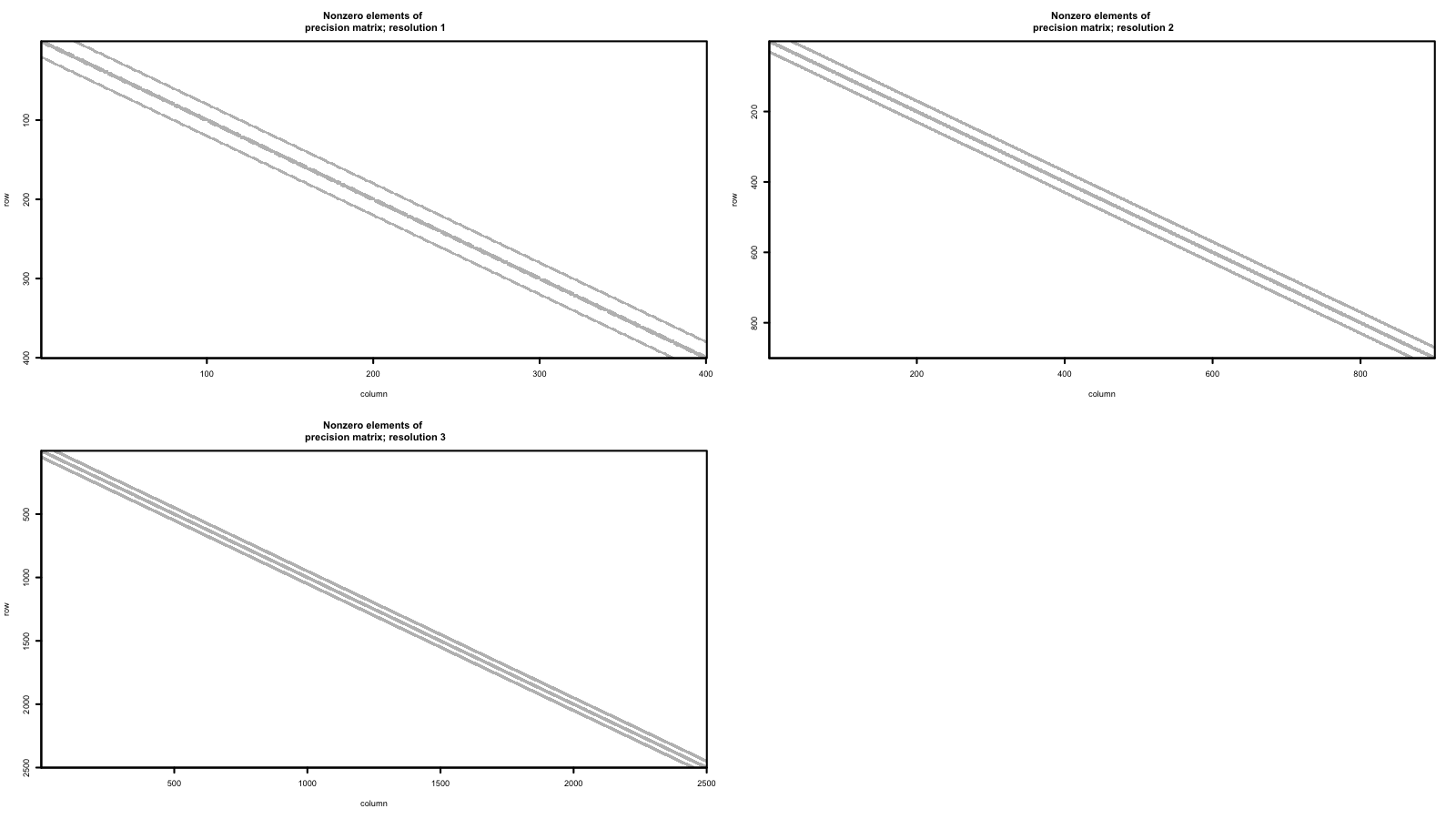In this Vignette, we demonstrate how to fit Bayesian spatial models using the multi-resolution model using Markov Chain Monte Carlo (MCMC).

# Defining the MRA model

This package provides a Bayesian implementation of the multi-resolution Gaussian process model presented by Nychka et al. (2015). This package is similar to the R package LatticeKrig::LatticeKrig (Nychka et al. (2016)) and provides an implementation that is suitable for use in MCMC samplers.

The model that the function mcmc_mra() fits is defined below. Let $$\mathbf{s}$$ be a spatial location in a domain of interest $$\mathcal{D}$$ and let $$y(\mathbf{s})$$ be the observation of the spatial process at location $$\mathbf{s}$$. Then, we can write the observation as

\begin{align*} y(\mathbf{s}) & = \mathbf{x}(\mathbf{s})' \boldsymbol{\beta} + \eta(\mathbf{s}) + \varepsilon(\mathbf{s}), \end{align*}

where $$\mathbf{x}(s)$$ is a vector of covariates at site $$\mathbf{s}$$, $$\boldsymbol{\beta}$$ are regression coefficients, $$\boldsymbol{\eta}(\mathbf{s})$$ is the realization of a correlated Gaussian process at location $$\mathbf{s}$$ and $$\varepsilon(\mathbf{s})$$ is the realization of an uncorrelated Gaussian error process. The model can be written in matrix form where

\begin{align*} \mathbf{y} & = \mathbf{X} \boldsymbol{\beta} + \boldsymbol{\eta} + \boldsymbol{\varepsilon}. \end{align*}

In matrix form, the spatially correlated random process has the distribution $$\boldsymbol{\eta} \sim \operatorname{N}(\mathbf{0}, \mathbf{C})$$ where the $$\mathbf{C}_{ij}$$ (the $$i$$th row and $$j$$th column of $$\mathbf{C}$$) is defined as the output of the covariance function $$cov(\mathbf{s}_i, \mathbf{s}_j)$$ at sites $$\mathbf{s}_i$$ and $$\mathbf{s}_j$$. The residual process $$\boldsymbol{\varepsilon} \sim \operatorname{N}(\mathbf{0}, \sigma^2 \mathbf{I})$$ models measurement error, commonly referred to as the nugget in the spatial statistics literature.

Rather than specifying a parametric form for the covariance function $$cov(\mathbf{s}_i, \mathbf{s}_j)$$, we approximate the covariance function effect with a multi-resolution approximation. This approximation gives $$\boldsymbol{\eta} \approx \sum_{m=1}^M \mathbf{W}_m \boldsymbol{\alpha}_m$$ where $$\mathbf{W}_m$$ is the Wendland basis expansion at the $$m$$th resolution and $$\boldsymbol{\alpha}_m$$ are the $$m$$th resolution random effect.

# Simulating data from the model

First, we simulate some data from the process of interest. We simulate a spatially explicit set of fired effects X that represent spatial variables like elevation, latitude, etc. Next, the spatial process is initialized

set.seed(11)

N <- 40^2

## setup the spatial process
locs <- as.matrix(
expand.grid(
seq(0, 1, length.out = sqrt(N)),
seq(0, 1, length.out = sqrt(N))
)
)
D <- fields::rdist(locs)

## fixed effects include intercept, elevation, and latitude
X <- cbind(1, as.vector(mvnfast::rmvn(1, rep(0, N), 3 * exp(-D / 20))), locs[, 2])
p <- ncol(X)

beta <- rnorm(ncol(X))

## MRA spatio-temporal random effect
M <- 3
n_coarse <- 10

MRA    <- mra_wendland_2d(locs, M = M, n_coarse = n_coarse, use_spam = TRUE)

# MRA    <- mra_wendland_2d(locs, M = M, n_max_fine_grid = 2^8, use_spam = TRUE)
W_list   <- MRA$W n_dims <- rep(NA, length(W_list)) dims_idx <- NULL for (i in 1:M) { n_dims[i] <- ncol(W_list[[i]]) dims_idx <- c(dims_idx, rep(i, n_dims[i])) } W <- do.call(cbind, W_list) Q_alpha <- make_Q_alpha_2d(sqrt(n_dims), rep(0.999, length(n_dims)), prec_model = "CAR") tau2 <- 10 * 2^(2 * (1:M - 1)) Q_alpha_tau2 <- make_Q_alpha_tau2(Q_alpha, tau2) ## initialize the random effect ## set up a linear constraint so that each resolution sums to one A_constraint <- sapply(1:M, function(i){ tmp = rep(0, sum(n_dims)) tmp[dims_idx == i] <- 1 return(tmp) }) a_constraint <- rep(0, M) alpha <- as.vector(rmvnorm.prec.const(n = 1, mu = rep(0, sum(n_dims)), Q = Q_alpha_tau2, A = t(A_constraint), a = a_constraint)) sigma2 <- runif(1, 0.25, 0.5) y <- X %*% beta + W %*% alpha + rnorm(N, 0, sqrt(sigma2)) Next, the sum-to-one constraint is verified by checking that within each dimension the sum of the $$\boldsymbol{\alpha}_m$$ parameters is 0. sum(alpha) ##  1.98782e-14 sum(alpha[dims_idx == 1]) ##  1.450229e-15 sum(alpha[dims_idx == 2]) ##  4.22995e-14 sum(alpha[dims_idx == 3]) ##  -2.387153e-14 ## Plotting the simulated data To explore the structure of the precision matrix of the spatial random effects, we plot the pattern of nonzero elements in the precision matrices $$\mathbf{Q}_{m}$$ for each resolution $$m$$. The sparse, banded structure in the precision matrices at each resolution are what enable efficient computation. ## plot Q_alpha_tau2 structure ## note: the display function gives a warning about the default cex setting layout(matrix(1:4, 2, 2, byrow = TRUE)) for (m in 1:M) { display(Q_alpha_tau2[which(dims_idx == m), which(dims_idx == m)]) title(main = paste("Nonzero elements of \n precision matrix; resolution", m)) }The MRA approach requires a set of grid point at each resolution. The set of grid points at each resolution extends beyond the spatial domain that is highlighted in the black box. data.frame( x = unlist(sapply(1:M, function(i) MRA$locs_grid[[i]][, 1])),
y = unlist(sapply(1:M, function(i) MRA$locs_grid[[i]][, 2])), resolution = factor(unlist(sapply(1:M, function(i) rep(i, each = nrow(MRA$locs_grid[[i]])))))
) %>%
ggplot(aes(x = x, y = y, color = resolution)) +
geom_point(alpha = 0.75, size = 0.75) +
ggtitle("MRA grid points") +
theme_bw() +
scale_colour_viridis_d(end = 0.8) +
geom_rect(
data = NULL,
aes(xmin = min(locs[, 1]),
xmax = max(locs[, 1]),
ymin = min(locs[, 2]),
ymax = max(locs[, 2])
),
fill  = NA,
color = "black",
alpha = 0.5
) +
theme_custom()